# Code Generation

The updated CodeGeneration package offers new support for translating to Python and Perl, expanded support for MATLAB®, and more options for controlling output.

The new Code Generation Assistant offers a convenient graphical interface to the CodeGeneration package.

### CodeGeneration[Python]

The new Python translator generates code in the Python 3 language for standalone execution. Similar to CodeGeneration's other translators, with CodeGeneration[Python], you can translate expressions to code fragments:cg = math.sqrt(a ** 2 + b ** 2 + c ** 2)

You can also translate procedures and larger programs.import sympy

 def cg0 (m):    r = 0    for i in range(1, m + 1):        r = r + sympy.prime(i)    return(r)

In addition to supporting the core Python 3 language, CodeGeneration[Python] also translates many Maple data structures and functions, including many routines for linear algebra and special functions, to equivalents in the popular numpy and scipy libraries for scientific computation.

#### Maple Object

Generated Python Outputcg25 = numpy.mat([[-59,-68,16,-95],[12,-67,9,-20],[-62,22,99,-25],[-33,14,60,51]])cg26 = -v ** 3 + 3 * v + 1 if v < 1 else 2 * v ** 3 - 9 * v ** 2 + 12 * v - 2 if v < 2 else -v ** 3 + 9 * v ** 2 - 24 * v + 22import numpy cg27 = lambda M,n: M - x * numpy.eye(n)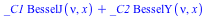cg28 = _C1 * scipy.special.jv(nu, x) + _C2 * scipy.special.yv(nu, x)cg29 = scipy.special.lambertw(math.pi + math.e, 100)

### CodeGeneration[Perl]

The new Perl translator allows you to prototype code in Maple for later inclusion in existing code in the Perl 5 language.
Since Maple, Perl, and Python are all loosely-typed interpreted programming languages, Maple programs can often be expressed very naturally in either Perl or Python.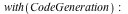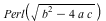\$cg30 = sqrt(-4 * \$a * \$c + \$b ** 2);

In particular, many of the advanced tools for string processing in Maple can be translated to Perl equivalents.  The following Maple code uses the StringTools[RegMatch] command for string matching with regular expressions.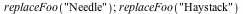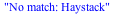The Perl language also has extensive support for regular expressions and CodeGeneration[Perl] makes use of this fact in its translation: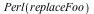#!/usr/bin/perl
 sub replaceFoo {  local(\$s) = @_;  if ((\$s =~ m/N[aeiou]*dl[ae]*/)) {    return("Match");  } else {    return("No match: " . \$s);  } }

In addition to supporting new language targets, Maple 18 includes expanded support for some existing targets and new options for controlling output.

The Matlab translator now provides MATLAB® equivalents for a larger set of Maple functions, including commands in the LinearAlgebra and ArrayTools packages.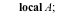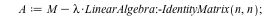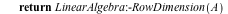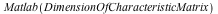function cg = DimensionOfCharacteristicMatrix(M, n, lambda)
 A = M - lambda * eye(n, n);  cg = size(A,1);

In addition, the new libraryorder option allows the user to specify a preference on which standard libraries are used for translation in the case when matching translations are available from multiple libraries.  This example from Python shows first a translation using both numpy.linalg and scipy.linalg libraries.import numpy.linalg
 import scipy.linalg def HilbertDeterminant (M, n):    return(numpy.linalg.det(scipy.linalg.hilbert(n)))

Typically, translating the determinant function  to numpy.linalg is the preferable choice.
In this case, because scipy.linalg library is already being used and contains its own implementation of the determinant function, it is preferable to use that implementation. This may be achieved using the libraryorder option to specify the relative weight to assign to each library.import scipy.linalg

 def HilbertDeterminant (M, n):    return(scipy.linalg.det(scipy.linalg.hilbert(n)))

## Ready for the next step?

*Evaluations are not available for students or personal edition users.